• ( 2 ) 偏序关系 等价关系 ( 等价关系 用于分类 | 偏序关系 用于组织 ) 2. 偏序集定义 ( 1 ) 偏序集定义 二. 偏序关系 示例 1. 小于等于关系 ( 1 ) 小于等于关系 说明 ( 2 ) 小于等于关系 分析 2. 大于等于...
文章目录一. 偏序关系1. 偏序关系定义( 1 ) 偏序关系定义 ( 自反 | 反对称 | 传递 )( 2 ) 偏序关系 与 等价关系 ( 等价关系 用于分类 | 偏序关系 用于组织 )2. 偏序集定义( 1 ) 偏序集定义二. 偏序关系 示例1. 小于等于关系( 1 ) 小于等于关系 说明( 2 ) 小于等于关系 分析2. 大于等于关系( 1 ) 大于等于关系 说明( 2 ) 大于等于关系 分析3. 整除关系( 1 ) 整除关系 说明( 2 ) 整除关系 分析4. 包含关系( 1 ) 包含关系 说明( 2 ) 包含关系 分析5. 加细关系( 1 ) 加细关系 说明( 2 ) 加细关系 分析

一. 偏序关系

1. 偏序关系定义

( 1 ) 偏序关系定义 ( 自反 | 反对称 | 传递 )

偏序关系 定义 :

1.前置条件 1 : $A \not= \varnothing$ , 并且 $R \subseteq A \times A$ ;

2.前置条件 2 : 如果 $R$ 是 自反 , 反对称 , 传递的 ;

① 自反 : 每个元素 自己 和 自己 都有关系 , $xRx$ ;
② 反对称 : 如果 $xRy$ 并且 $yRx$ 则 $x=y$ , 即 $x \not=y$ ,  $xRy$ 和 $yRx$ 不能同时存在 ; 可以没有 , 但是一定不能同时出现 ;
③ 传递 : 如果 有  $xRy$ ,  $yRz$ , 那么必须有  $xRz$ , 如果前提不成立 , 那么也勉强称为传递 ;

3.结论 : 称 $R$ 为 $A$ 上的偏序关系 ;

4.表示 : 使用 $\preceq$ 表示偏序关系 ;

5.读法 : $\preceq$ 读作 "小于等于" ;

6.使用公式表示 :
$<x, y> \in R \Longleftrightarrow xRy \Longleftrightarrow x \preceq y$

7.公式解读 : 如果 $x$ , $y$ 两个元素 构成 有序对 $<x,y>$ , 并且在偏序关系$R$ 中 , $x$ 和 $y$ 具有 $R$ 关系 , 也可以写成 $x$ 小于等于 ( 偏序符号 ) $y$ ;

8.常见的偏序关系 : 树 上 的 小于等于关系 , 集合上的包含关系 , 非 $0$ 自然数之间的整除关系 , 都是常见的偏序关系 ;

( 2 ) 偏序关系 与 等价关系 ( 等价关系 用于分类 | 偏序关系 用于组织 )

偏序关系 与 等价关系 :

1.表示层次结构 : 偏序关系是非常常用的二元关系 , 通常用来 表示 层次结构 ;
2.等价关系 : 等价关系 是 用来分类的 , 将一个 集合 分为 几个等价类 ;
3.偏序关系 : 偏序关系 通常是 用来组织的 , 在每个类的内部 , 赋予其一个结构 , 特别是层次结构 , 有上下层级 ,

2. 偏序集定义

( 1 ) 偏序集定义

偏序集 定义 :

1.前置条件 1 : $\preceq$ 是 $A$ 上的 偏序关系 ;
2.结论 : $<A , \preceq>$ 是偏序集 ;
3.解读 : 集合 $A$ 与 偏序关系 $\preceq$ 构成的有序对 , 称为 偏序集 ;

二. 偏序关系 示例

1. 小于等于关系

( 1 ) 小于等于关系 说明

偏序集示例 1 ( 小于等于关系 $\leq$ 是 偏序关系 ) :

1.公式表示 : $\varnothing \not= A \subseteq R , <A , \leq >$
2.语言描述 : 如果 $A$ 是 实数集 $R$ 的 子集 , 并且 $A$ 不能 是 空集 $\varnothing$ ,  集合 $A$ 中的 小于等于关系 , 是偏序关系 ;
3.使用集合形式表示关系 : $\leq = \{ <x,y> | x,y \in A \land x \leq y \}$

( 2 ) 小于等于关系 分析

实数集 $A$ 上的 小于等于关系 ( $\leq$ ) 分析 :

1.自反性质分析 : $x$ 小于等于 $x$ , $x \leq x$ , 是成立的 , 小于等于关系 是 自反的 ;
2.反对称性质分析 : $x$ 小于等于 $y$ , $y$ 小于等于 $x$ , 推出 $x = y$ , 符合 反对称性质 的 定义 , 因此 小于等于 关系 是 反对称的 ,
3.传递性质分析 : $x$ 小于等于 $y$ , $y$ 小于等于 $z$ , $x$ 小于等于 $z$ , 是成立的 , 因此 小于等于关系 是 传递的 ;
4.总结 : 综上所述 , 小于等于 关系 是 偏序关系 ;

2. 大于等于关系

( 1 ) 大于等于关系 说明

偏序集示例 2 ( 大于等于关系 $\geq$  是 偏序关系 ) :

1.公式表示 : $\varnothing \not= A \subseteq R , <A , \geq >$
2.语言描述 : 如果 $A$ 是 实数集 $R$ 的 子集 , 并且 $A$ 不能 是 空集 $\varnothing$ ,  集合 $A$ 中的 大于等于关系 ( $\geq$ ) , 是偏序关系 ;
3.使用集合形式表示关系 : $\geq = \{ <x,y> | x,y \in A \land x \geq y \}$

( 2 ) 大于等于关系 分析

实数集 $A$ 上的 大于等于关系 ( $\geq$ ) 分析 :

1.自反性质分析 : $x$ 大于等于 $x$ , $x \geq x$ , 是成立的 , 大于等于关系 是 自反的 ;
2.反对称性质分析 : $x$ 大于等于 $y$ , $y$ 大于等于 $x$ , 推出 $x = y$ , 符合 反对称性质 的 定义 , 因此 大于等于 关系 是 反对称的 ,
3.传递性质分析 : $x$ 大于等于 $y$ , $y$ 大于等于 $z$ , $x$ 大于等于 $z$ , 是成立的 , 因此 大于等于关系 是 传递的 ;
4.总结 : 综上所述 , 大于等于 关系 是 偏序关系 ;

3. 整除关系

( 1 ) 整除关系 说明

偏序集示例 3 ( 整除关系 是 偏序关系 ) :

1.公式表示 : $\varnothing \not= A \subseteq Z_+ = \{ x | x \in Z \land x > 0 \}<A , | >$
2.语言描述 : 如果 $A$ 是 正整数集 $Z_+$ 的 子集 , 并且 $A$ 不能 是 空集 $\varnothing$ ,  集合 $A$ 中的 整除关系 ( $|$ ) , 是偏序关系 ;
3.使用集合形式表示关系 : $|= \{ <x,y> | x,y \in A \land x | y \}$
4.整除关系 : $x|y$ , $x$ 是 $y$ 的因子 , 或 $y$ 是 $x$ 的倍数 ;

( 2 ) 整除关系 分析

正整数集 $A$ 上的 整除关系 ( $|$ ) 分析 :

1.自反性质分析 : $x$ 整除 $x$ , $x | x$ , 是成立的 , 整除关系 ( | ) 是 自反的 ;
2.反对称性质分析 : $x$ 整除 $y$ , $y$ 整除 $x$ , 两个正整数互相都能整除 , 它们只能相等 , 推出 $x = y$ , 符合 反对称性质 的 定义 , 因此 整除 关系 是 反对称的 ,
3.传递性质分析 : $x$ 整除 $y$ , $y$ 整除 $z$ , $x$ 整除 $z$ , 是成立的 , 因此 整除关系 是 传递的 ;
4.总结 : 综上所述 , 整除 关系 是 偏序关系 ;

4. 包含关系

( 1 ) 包含关系 说明

偏序集示例 4 ( 包含关系 $\subseteq$ 是 偏序关系 ) :

1.公式表示 : $\mathscr{A} \subseteq P(A) , \subseteq = \{<x , y> | x , y \in \mathscr{A} \land x \subseteq y \}$
2.语言描述 : 集合 $A$ 上的幂集合 $P(A)$ ,  $P(A)$ 的子集合 构成 集族 $\mathscr{A}$ , 该集族 $\mathscr{A}$ 上的包含关系 , 是偏序关系 ;

( 2 ) 包含关系 分析

分析 集合的  子集族 之间的包含关系 :

① 假设一个比较简单的集合
$A=\{a, b\}$

② 分析 下面 $A$ 的 3 个子集族 ;
$\mathscr{A}_1 = \{ \varnothing , \{a\} , \{b\} \}$
集族 $\mathscr{A}_1$ 包含 空集 $\varnothing$ , 单元集 $\{a\}$ , 单元集 $\{b\}$  ;
$\mathscr{A}_2 = \{ \{a\} , \{a, b\} \}$
集族 $\mathscr{A}_2$ 包含 单元集 $\{a\}$ , 2 元集 $\{a, b\}$ ;
$\mathscr{A}_3 = P(A) = \{ \varnothing , \{a\} , \{b\} , \{a, b\} \}$
集族 $\mathscr{A}_3$ 包含 空集 $\varnothing$ , 单元集 $\{a\}$ ,  单元集 $\{b\}$ , 2 元集 $\{a, b\}$ ; 这是 集合 $A$ 的 幂集 ;

③ 列举出集族 $\mathscr{A}_1$ 上的包含关系  :
$\subseteq_1 = I_{\mathscr{A}1} \cup \{ <\varnothing , \{a\}> , <\varnothing , \{b\}> \}$
$\subseteq_1$ 是集合 $\mathscr{A}1$ 上的偏序关系 ;
即 分析 空集 $\varnothing$ , 单元集 $\{a\}$ , 单元集 $\{b\}$ 三个 集合之间的包含关系 :

1.恒等关系 $I_{\mathscr{A}1}$ : $<\{a\} , \{a\}> 和 <\{b\} , \{b\}>$ ,  集合上的恒等关系 , 每个集合 肯定 自己包含自己 ;
2.$<\varnothing , \{a\}>$ : 空集 肯定 包含于 集合 $\{a\}$ ;
3.$<\varnothing , \{b\}>$ : 空集 肯定 包含于 集合 $\{b\}$ ;
4.总结 : 这些包含关系 的性质分析 :

① 自反 : 每个元素自己 包含 自己 , $A \subseteq A$ , 包含关系具有 自反性质 ;
② 反对称 : 如果 集合 $A \subseteq B$ , $B \subseteq A$ , 那么 $A = B$ , 显然 包含关系 具有反对称性质 ;
③ 传递 : 如果 $A \subseteq B$ , 并且 $A \subseteq C$ , 那么有 $A \subseteq C$ , 包含关系 具有传递性质 ;

④ 列举出集族 $\mathscr{A}_2$ 上的包含关系  :
$\subseteq_2 = I_{\mathscr{A}2} \cup \{ <\{a\} , \{a, b\}>$
$\subseteq_2$ 是集合 $\mathscr{A}2$ 上的偏序关系 ;

⑤ 列举出集族 $\mathscr{A}_3$ 上的包含关系  :
$\subseteq_3 = I_{\mathscr{A}3} \cup \{ <\varnothing , \{a\}> , <\varnothing , \{b\}>, <\varnothing , \{a, b\}> , <\{a\} , \{a, b\}> , <\{b\} , \{a, b\}> \}$
$\subseteq_3$ 是集合 $\mathscr{A}_3$ 上的偏序关系 ;

5. 加细关系

( 1 ) 加细关系 说明

偏序集示例 5 ( 加细关系 $\preceq_{加细}$ 是 偏序关系 ) :

1.加细关系描述 : $A \not= \varnothing$ , $\pi$ 是 由 $A$ 的 一些划分 组成的集合 ;

$\preceq_{加细} = \{<x , y> | x , y \in \pi \land x 是 y 的 加细\}$

2.划分 : 划分 是 一个 集族 ( 集合的集合 ) , 其元素是集合 又叫 划分快 , 其中 每个元素(集族中的元素)集合 中的 元素 是 非空集合 $A$ 的元素 ;

① 该集族不包含空集 ;
② 该集族中任意两个集合都不想交 ;
③ 该集族中 所有 元素 取并集 , 得到 集合 $A$ ;

( 2 ) 加细关系 分析

分析 集合的  划分之间 的 加细 关系 :
① 集合 $A = \{a, b, c\}$ , 下面的 划分 和 加细 都基于 该 集合 进行分析 ;

② 下面 列出集合 $A$ 的 5 个划分 :
划分 1 : 对应 1 个等价关系 , 分成 1 类 ;
$\mathscr{A}_1 =\{ \{ a, b, c \} \}$
划分 2 :  对应 2 个等价关系 , 分成 2 类 ;
$\mathscr{A}_2 = \{ \{ a \} , \{ b, c \} \}$
划分 3 : 对应 2 个等价关系 , 分成 2 类 ;
$\mathscr{A}_3 = \{ \{ b \} , \{ a, c \} \}$
划分 4 : 对应 2 个等价关系 , 分成 2 类 ;
$\mathscr{A}_4 = \{ \{ c \} , \{ a, b \}\}$
划分 5 : 对应 3 个等价关系 , 分成 3 类 ; 每个元素自己自成一类
$\mathscr{A}_5 = \{ \{ a \} , \{ b \}, \{ c \} \}$
③ 下面 列出要分析的几个由划分组成的集合 :
集合 1 :
$\pi_1 = \{ \mathscr{A}_1, \mathscr{A}_2 \}$
集合 2 :
$\pi_2 = \{ \mathscr{A}_2, \mathscr{A}_3 \}$
集合 3 :
$\pi_3 = \{ \mathscr{A}_1, \mathscr{A}_2, \mathscr{A}_3, \mathscr{A}_4, \mathscr{A}_5 \}$
④ 集合 $\pi_1$ 上的加细关系分析 :

1.自己是自己的加细 : 每个划分 , 自己是自己的加细 , 因此 加细关系中 有 $I_{\pi 1}$ , $<\mathscr{A}_1 , \mathscr{A}_1>$ , $<\mathscr{A}_2 , \mathscr{A}_2>$ ;
2.其它加细关系 : $\mathscr{A}_2$ 划分中的 每个划分块 , 都是 $\mathscr{A}_1$ 划分 中块 的某个划分块的子集合 , 因此有 $\mathscr{A}_2$ 是 $\mathscr{A}_1$ 的加细 , 记做 $<\mathscr{A}_2, \mathscr{A}_1>$  ;
3.加细的定义 : $\mathscr{A}_1$ 和 $\mathscr{A}_2$ 都是集合 $A$ 的划分,  $\mathscr{A}_2$ 中的 每个划分块 , 都含于 $\mathscr{A}_1$ 中的某个划分块中 , 则称 $\mathscr{A}_2$ 是 $\mathscr{A}_1$ 的加细 ;

- 4.加细关系列举 :
$\preceq_1 = I_{\pi 1} \cup \{ <\mathscr{A}_2, \mathscr{A}_1> \}$

⑤ 集合 $\pi_2$ 上的加细关系分析 :

1.自己是自己的加细 : 每个划分 , 自己是自己的加细 , 因此 加细关系中 有 $I_{\pi 2}$ , $<\mathscr{A}_3 , \mathscr{A}_3>$ , $<\mathscr{A}_2 , \mathscr{A}_2>$ ;
2.其它加细关系 : $\mathscr{A}_2$ 和  $\mathscr{A}_3$ 这两个划分互相不是加细 , 因此 该集合中没有其它加细关系 ;

- 4.加细关系列举 :
$\preceq_2 = I_{\pi 2}$

⑥ 集合 $\pi_3$ 上的加细关系分析 :

1.自己是自己的加细 : 每个划分 , 自己是自己的加细 , 因此 加细关系中 有 $I_{\pi 3}$ , $<\mathscr{A}_1 , \mathscr{A}_1>$ , $<\mathscr{A}_2 , \mathscr{A}_2>$, $<\mathscr{A}_3 , \mathscr{A}_3>$, $<\mathscr{A}_4 , \mathscr{A}_4>$, $<\mathscr{A}_5 , \mathscr{A}_5>$ ;
2.其它加细关系 :

① 与 $\mathscr{A}_5$ 划分相关的加细 :  $\mathscr{A}_5$ 是划分最细的 等价关系 , $\mathscr{A}_5$ 是其它所有 划分 的加细 , 因此有 $<\mathscr{A}_5 , \mathscr{A}_4>$ , $<\mathscr{A}_5 , \mathscr{A}_3>$ , $<\mathscr{A}_5 , \mathscr{A}_2>$ , $<\mathscr{A}_5 , \mathscr{A}_1>$ ;
② 与 $\mathscr{A}_1$ 划分相关的加细 : $\mathscr{A}_1$ 是划分最粗的 等价关系 , 所有的划分 都是 $\mathscr{A}_1$ 的加细 , 因此有 $<\mathscr{A}_5 , \mathscr{A}_1>$ , $<\mathscr{A}_4 , \mathscr{A}_1>$ , $<\mathscr{A}_3 , \mathscr{A}_1>$ , $<\mathscr{A}_2 , \mathscr{A}_1>$ ;

4.加细关系列举 :

$\preceq_3 = I_{\pi 3} \cup \{ <\mathscr{A}_5 , \mathscr{A}_4> , <\mathscr{A}_5 , \mathscr{A}_3> , <\mathscr{A}_5 , \mathscr{A}_2> , <\mathscr{A}_5 , \mathscr{A}_1> , <\mathscr{A}_4 , \mathscr{A}_1>, <\mathscr{A}_3 , \mathscr{A}_1>, <\mathscr{A}_2 , \mathscr{A}_1> \}$


展开全文偏序集
• b）用例用例之间的关系包含关系（include）、扩展关系（extend）、泛化关系。 1.包含关系 包含关系描述的是一个用例需要某种功能，而该功能被另外一个用例定义，那么在用例的执行过程中，就可以调用已经定义好...
一、用例图中的各种关系

a）参与者与用例间的关联关系：参与者与用例之间的通信，也成为关联或通信关系。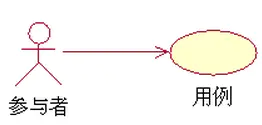b）用例与用例之间的关系：包含关系（include）、扩展关系（extend）、泛化关系。

1.包含关系

包含关系描述的是一个用例需要某种功能，而该功能被另外一个用例定义，那么在用例的执行过程中，就可以调用已经定义好的用例。表示符号：<<include>>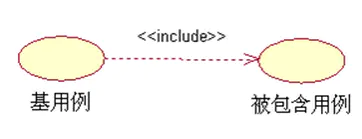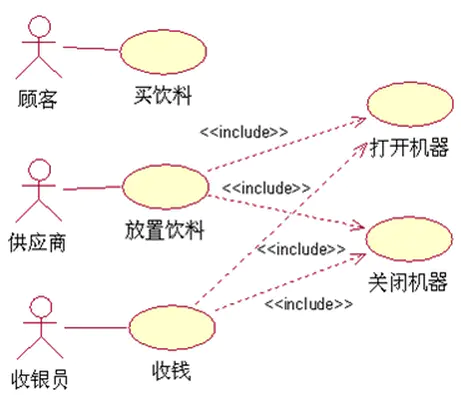实例一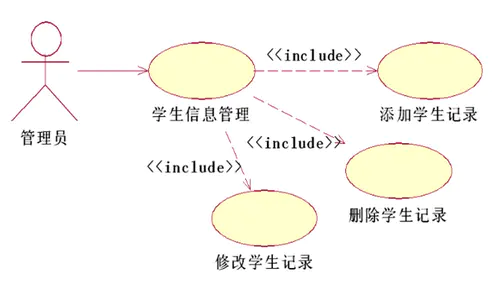实例二

2.扩展关系

用一个用例（可选）扩展另一个用例（基本例）的功能，将一些常规的动作放在一个基本用例中，将可选的或只在特定条件下才执行的动作放在它的扩展用例中。表示符号：<<extend>>。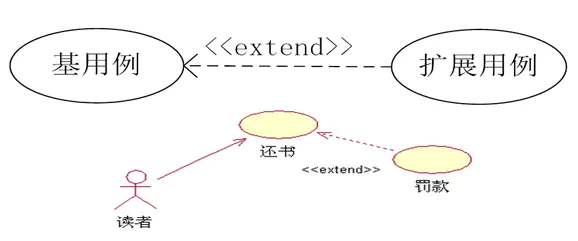3.泛化关系

子用例继承了父用例所有的结构、行为和关系，是父用例的一种特殊形式。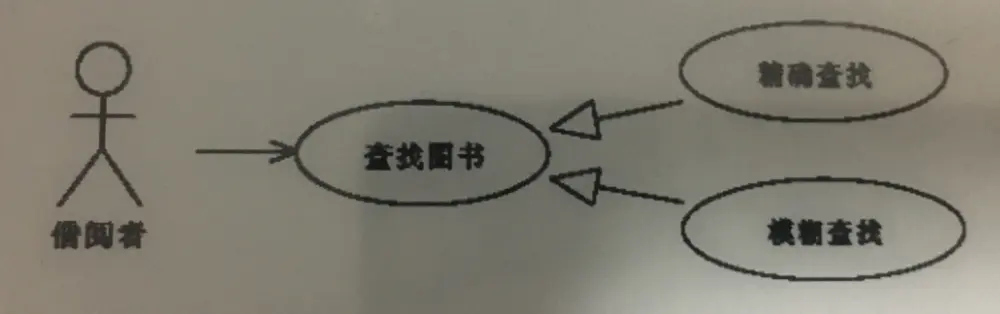展开全文• 包含关系：通常是指一个大的用例包含了几个小的用例，几个小的用例组成一个大的用例； 扩展关系：两个独立的用例，完成一个后可以开启另一个，两个用例本身都是独立的。 例如：用户管理的用例包括用户添加和用户删除...
 包含关系：通常是指一个大的用例包含了几个小的用例，几个小的用例组成一个大的用例； 扩展关系：两个独立的用例，完成一个后可以开启另一个，两个用例本身都是独立的。 例如：用户管理的用例包括用户添加和用户删除；用户登陆用例后可以进行用例退出。
展开全文扩展
• 一、哈斯图示例 ( 整除关系 ) 、 二、哈斯图示例 ( 包含关系 ) 、 三、哈斯图示例 ( 加细关系 ) 、
文章目录一、哈斯图示例 ( 整除关系 )二、哈斯图示例 ( 包含关系 )三、哈斯图示例 ( 加细关系 )

一、哈斯图示例 ( 整除关系 )

集合 $A = \{ 1, 2, 3, 4, 5, 6, 9, 10, 15 \}$ ,
集合 $A$ 上的整除关系 “$|$” 是偏序关系 ,
偏序集是 $$

$x$ 整除 $y$ , $x$ 是除数 (分母) , $y$ 是被除数 (分子) ; $\dfrac{y}{x}$
$y$ 能被 $x$ 整除 , $x$ 是除数 (分母) , $y$ 是被除数 (分子) ; $\dfrac{y}{x}$

绘制上述偏序集的哈斯图 :$1$ 是最小的 , $1$ 能整除所有的数 ;
$1$ 上面的一层是素数 , 素数只能被 $1$ 和其本身整除 ; 素数肯定是覆盖 $1$ 的 ; 即素数与 $1$ 之间没有元素 ;

素数之上的数 , 由素数相乘的数组成 ;
$6$ 既可以整除 $2$ , 又可以整除 $3$ , 因此其既覆盖 $2$ , 又覆盖 $3$ ;
$10$ 既可以整除 $2$ , 又可以整除 $5$ , 因此其既覆盖 $2$ , 又覆盖 $5$ ;
$15$ 既可以整除 $3$ , 又可以整除 $5$ , 因此其既覆盖 $3$ , 又覆盖 $5$ ;
$4$ 可以整除 $2$ , 因此 $4$ 覆盖 $2$ ;
$9$ 可以整除 $3$ , 因此 $9$ 覆盖 $3$ ;

二、哈斯图示例 ( 包含关系 )

集合 $A = \{ a, b , c \}$ ,
集族 $\mathscr{A}$ 包含于 $A$ 集合的幂集 , $\mathscr{A} \subseteq P(A)$ ,
集族 $\mathscr{A} = \{ \varnothing , \{ a \} , \{ b \} , \{ c \} , \{ a , b \} , \{ b,c \} , \{ a, c \} \}$
集族 $\mathscr{A}$ 上的 包含关系 “$\subseteq$” 是偏序关系 ,
偏序集是 $<\mathscr{A} , \subseteq >$空集 包含于 所有集合 , 是最小的 , 在哈斯图最下面 ;
空集 之上是单元集 , 单元集  覆盖 空集 , 它们之间并不会有第三个元素 ;
三个单元集之间相互没有包含关系 , 是不可比的 ;
单元集 之上是 双元集 , 每个 双元集 之下就是其包含的对应的单元集 ;

三、哈斯图示例 ( 加细关系 )

加细关系 是 有序对集合 , 其中每个 有序对的元素 是 集族 ;

集合 $A$ 非空 , $\pi$ 是 $A$ 集合划分组成的集合 , 每个划分都是一个集族 ;
划分参考 : 【集合论】划分 ( 划分 | 划分示例 | 划分与等价关系 )
集族之间有一种关系 , 加细关系 , 使用符号 $\preccurlyeq_{加细}$ 表示 ;
加细关系 $\preccurlyeq_{加细}$ 符号化表示 :
$\preccurlyeq_{加细} = \{  | x, y \in \pi \land x 是 y 的加细 \}$

前提 :

集合 $A = \{ a, b , c , d \}$

集族 $\mathscr{A}_1= \{ \{ a \} , \{ b \} , \{ c \} , \{ d \} \}$

集族 $\mathscr{A}_2 = \{ \{ a , b \} , \{ c , d \} \}$

集族 $\mathscr{A}_3= \{ \{ a,c \} , \{ b,d\} \}$

集族 $\mathscr{A}_4= \{ \{ a \} , \{ b, c , d \} \}$

集族 $\mathscr{A}_5= \{ \{ a \} , \{ b \} , \{ c , d \} \}$

集族 $\mathscr{A}_6 = \{ \{ a , b , c , d\} \}$

上述集族都是 $A$ 集合的划分 ;

划分关系的哈斯图 :$\mathscr{A}_1$ 是所有划分的加细 , 是最细的划分 , 在哈斯图最下面 ;
所有的划分都是 $\mathscr{A}_6$ 的加细 , 是最粗粒度的划分, 在哈斯图最上面 ;
$\mathscr{A}_5$ 既是 $\mathscr{A}_2$ 的加细 , 又是 $\mathscr{A}_4$ 的加细 ;
$\mathscr{A}_3$ 与 $\mathscr{A}_4$ 互相不是对方的加细 , 不可比 ;
$\mathscr{A}_2$ 与 $\mathscr{A}_4$ 互相不是对方的加细 , 不可比 ;
$\mathscr{A}_2$ 与 $\mathscr{A}_3$ 互相不是对方的加细 , 不可比 ;
$\mathscr{A}_3$ 与 $\mathscr{A}_5$ 互相不是对方的加细 , 不可比 ;


展开全文哈斯图 覆盖
• 目录UML定义UML图用例图定义用例图中的事物用例图中的关系关联关系包含关系特点使用场景扩展关系特点使用场景泛化关系特点使用场景 UML定义 UML（Unified Modeling Language，统一建模语言），是一种面向对象的建模...uml uml类图
• 用例元素包括参与者和用例，用例间的关系主要是：继承关系、扩展关系包含关系，这里比较难区分的的是扩展关系包含关系，比较容易混淆，分析整理一下。 【知识点】 扩展关系（Extend）：当某个新用例在原来的...
• ChinesePersonRelationGraph ...中文人物关系知识图谱项目,内容包括中文人物关系图谱构建,基于知识库的数据回标,基于远程监督bootstrapping方法的人物关系抽取,基于知识图谱的知识问答等应用. 项目地址:htt...知识图谱
• UML 用例图_包含关系和扩展关系和泛化关系 1.包含关系是从基本用例到包含用例的关系， 它指定如何将为包含用例定义的行为明确地插入到为基本用例定义的行为中。 示例： 在 ATM 系统中，用例 Withdraw Cash（提款...扩展 uml 任务
• 概述 传统的集合运算 （并，差，交，笛卡尔积） 专门的关系运算并（Union）R和S 具有相同的目n（即两个关系都有n个属性） 相应的属性取自同一个域R∪S 仍为n目关系，由属于R或属于...仍为n目关系，由属于R而不属于数据库 union 属性
• 字符串集合中相互包含关系算法 先介绍下使用场景，源文件是一个excel表格，要根据某一列的值生成正则表达式，为保证正则表达式的准确性，被包含的数据肯定是不能直接生成正则表达式的，例如，产科和妇产科，如果...python 算法
• // 三层四层均分第二层角度 if(i==3){ //第四层开始弧度为第三层的结束角度 start = array / total * 2 * Math.PI + 1.2 * Math.PI; //第四层结束弧度为 第四层以0为开始的结束角度+第三层的结束角度 ...微信小程序
• 介是你没有玩过的船新版本包含最小依赖集求法候选码求法 在模式分解之前，首先对于1NF,2NF,3NF,BCNF做一个简明扼要的介绍。 1NF是指数据库表的每一列都是不可分割的基本数据项，即实体中的某个属性不能有多个值...BCNF 数据库 最小依赖集
• 一、 特殊关系 、 二、 集合上的特殊关系 、 三、 整除关系 、 四、 大小关系
• ③设A,B,C∈P(X),如果A⊆B且B⊆C,，则A⊆C,所以⊆满足传递性transitive.（至此可以说明⊆是一个偏序关系，(P(X),⊆)是一个偏序集） ④设A,B属于偏序集(P(X),⊆)，因为A⊆A∪B,B⊆A∪B，如果A⊆C,B⊆C,那么A∪B⊆C，...算法
• Python List 包含关系判定 网上没找到合适的代码来判断一个list是否完全包含另一个list，自已写了一个函数。 ## 比较两个列表A和B是否A包含于B，可以比较有间隔的列表 def allin(A, B): A.sort() B.sort() if len...python
• 等价关系与等价类的例题 商集的定义 集合中的三种关系 等价关系：设R为定义在集合A上的关系，若R是自反的，对称的，传递的，则R称为等价关系 相容关系：设R为定义在集合A上的关系，若R是自反的和对称的，则R称为...
• 实体(记录)，意为对于用户来说非常重要、需要在数据库中表示的内容，关系表：数据以关系(relation)的形式来存储，具有以下特征： 1、表的每行存储了某个实体或实体某个部分的数据。 2、表的每列包含了用于表示实体...数据库
• 用例关系 用例图使用户 开发人员交流的一种重要的方式，是对用户需求的一种描述。开发人员从用户的角度整体上理解系统的功能。 1）用例“注册学生信息”和“充值”用例...包含关系中基本用例的基本流执行时，...uml
• 关系数据库关系型数据库一、相关概念 一、相关概念 ●关系型数据库: 关系型数据库是一个结构化的数据库,创建在关系模型（二维表格模型）基础上,一般面向于记录。 SQL语句(标准数据查询语言）就是一种基于关系型...数据库 mysql nosql
• 在 IntelliJ IDEA 中这个查看一个类也就是当前类的所有继承关系包括实现的所有的接口和继承的类， 这个继承，不仅仅是一级的继承关系包括好几层的继承。父类的父类的父类。直到最后。 可以很清楚明了的了解一个...实现接口 diagram
• 所以学习了一下，发现非关系型数据库最近非常的火，特别适用于某些应用如SNS网站，所以学习了NoSql我们常用的关系型数据库之间的差别。 总的来说 1.非关系型数据库不需要表表之间有联系。 2.非关系型数据库对...nosql 数据库
• 例如判断bill表中，detail字段中不包含money的结果集。 SELECT * FROM bill a WHERE !LOCATE(a.money,a.detail)；
• ## springboot与springcloud的关系

万次阅读 多人点赞 2019-07-08 19:47:20
方案2.1 首先说下maven2.2 springboot介绍2.2.1 介绍2.2.2 依赖图2.3 springcould介绍2.3.1 新建springcloud项目2.3.2 pom.xml文件2.3.3 依赖图2.4 关系总结 1. 问题描述 随着springboot、springcloud的不断迭代...springboot
• 关系模型的数据结构非常简单，只包含单一的数据结构——关系。在用户看来，关系模型中数据的逻辑结构是一张扁平的二维表。 1.1域 域是一组具有相同数据类型值的集合。 1.2笛卡儿积 笛卡儿积是域上的一种集合...数据库
• 今天突然遇到一个需求需要支持‘包含关系’的表达式，左思右想不知道怎么实现，先试了一下sql中的in，不行！！然后突然想到是否支持字符串包含函数contains()，试一下果然可以。 以下是我的关系表达式：\${“1,2,3”....
• mysql解决模糊查询包含关系 后台要根据期限筛选查询时如果用like， SELECT * from t_user_accord_invest t where t.invest_period like '%1%'; 就会出现 参数为1时 ，13的也能筛选出来，出现查询bug。 ...
• java中contains方法是判断是否存在包含关系的， 比如说集合a =[1,2,3,4]，b=1，那么a就包含b； contains返回的是布尔类型true 和false，包含的话就返回true，不包含的话就返回false 例： public class pratise...
• 基于深度学习的命名实体识别与关系抽取 摘要：构建知识图谱包含四个主要的步骤：数据获取、知识抽取、知识融合和知识加工。其中最主要的步骤是知识抽取。知识抽取包括三个要素：命名实体识别（NER）、实体关系抽取......